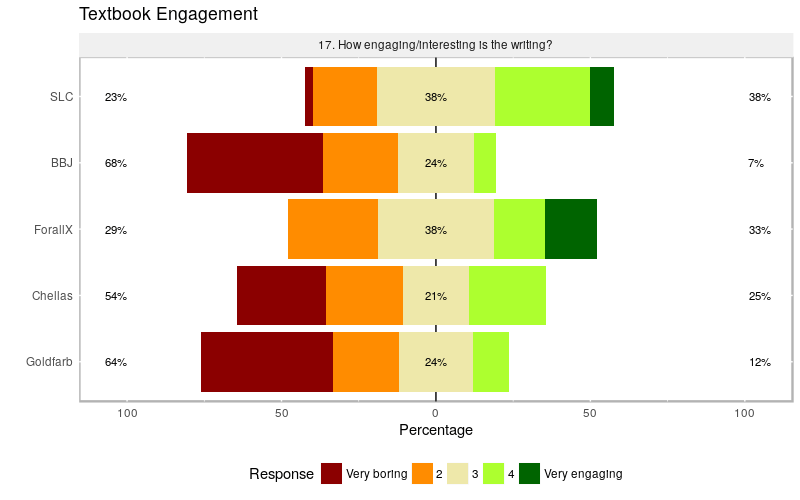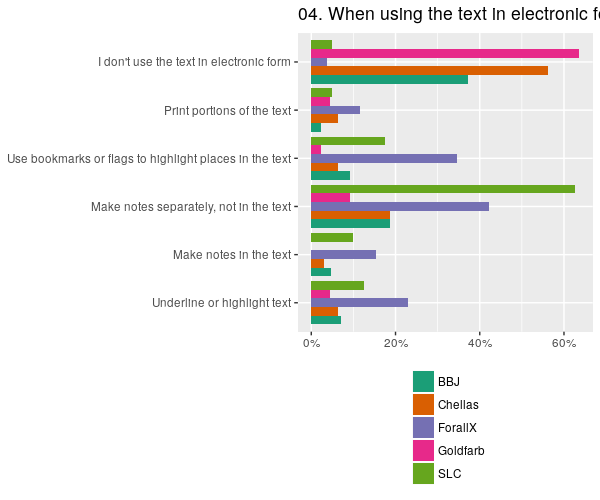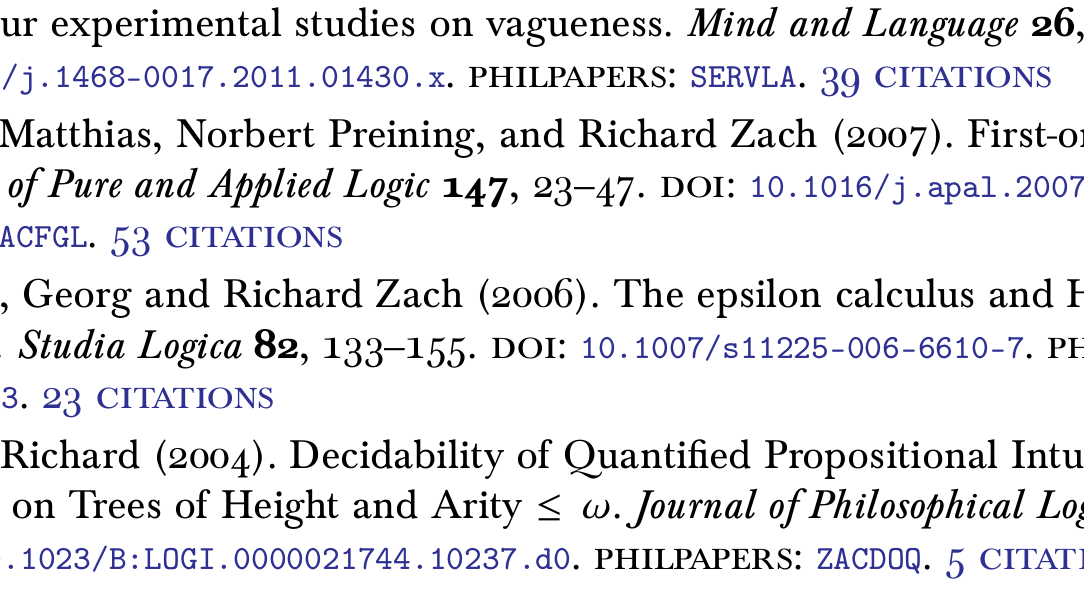# Graphing Survey Responses

As I reported last year, we’ve been running surveys in our classes that use open logic textbooks. We now have another year of data, and I’ve figured out R well enough to plot the results. Perhaps someone else is in a similar situation, so I’ve written down all the steps. Results aren’t perfect yet. All the data and code is on Github, and any new discoveries I make will be updated there.

What follows is the content of the HOWTO:

As part of two Taylor Institute Teaching & Learning Grants, we developed course materials for use in Calgary’s Logic I and Logic II courses. In the case of Logic I, we also experimented with partially flipping the course. One of the requirements of the grants was to evaluate the effectiveness of the materials and interventions. To evaluate the textbooks, we ran a survey in the courses using the textbooks, and in a number of other courses that used commercial textbooks. These surveys were administered through SurveyMonkey. To evaluate the teaching interventions, we designed a special course evaluation instrument that included a number of general questions with Likert responses. The evaluation was done on paper, and the responses to the Likert questions were entered into a spreadsheet.

In order to generate nice plots of the results, we use R. This documents the steps taken to do this.

## Installing R, RStudio, and likert

We’re running RStudio, a free GUI frontend to R. In order to install R on Ubuntu Linux, we followed the instructions here, updated for zesty:

• Start “Software & Updates”, select add a source, enter the line
http://cran.rstudio.com/bin/linux/ubuntu zesty/


Then in the command line:

$sudo apt-get install r-base r-base-dev  • We then installed RStudio using the package provided here. The R packages for analyzing Likert data and plotting them require devtools, which we installed following the instructions here: $ sudo apt-get install build-essential libcurl4-gnutls-dev libxml2-dev libssl-dev
$R > install.packages('devtools')  • Now you can install the likert package from Github: > install_github('likert', 'jbryer') ## Preparing the data The source data comes in CSV files, teachingevals.csv for the teaching evaluation responses, and textbooksurvey.csv for the textbook survey responses. Since we entered the teaching evaluation responses manually, it was relatively simple to provide them in a format usable by R. Columns are Respondent ID for a unique identifier, Gender (M for male, F for female, O for other), Major, Year, Q1 through Q9 for the nine Likert questions. For each question, a response of one of Strongly Agree, Agree, Neutral, Disagree, or Strongly Disagree is recorded. For the textbook survey we collected a whole lot of responses more, and the data SurveyMonkey provided came in a format not directly usable by R. We converted it to a more suitable format by hand. • SurveyMonkey results have two header lines, the first being the question, the second being the possible responses in multiple-response questions. We have to delete the second line. For instance, a question may have five different possible responses, which correspond to five columns. If a box was checked, the corresponding cell in a response will contain the answer text, otherwise it will be empty. In single-choice and Likert responses, SurveyMonkey reports the text of the chosen answer. For analysis, we wanted a simple 1 for checked and 0 for unchecked, and a number from 1 to 5 for the Likert answers. This was done easily enough with some formulas and search-and-replacing. • Since the question texts in the SurveyMonkey spreadsheet don’t make for good labels for importing from CSV, we replaced them all by generic labels such as Q5 (or Q6R2, for Question 6, Response 2, for multiple-choice questions). • We deleted data columns we don’t need such as timestamps and empty colums for data we didn’t collect such as names and IP addresses. • We added columns so we can collate data more easily: Section to identify the individual course the data is from, Course for which course it is (PHIL279 for Logic I, PHIL379 for Logic II), Term for Fall or Winter term, Open to distinguish responses from sections using an open or a commercial text, and Text for the textbook used. Text is one of SLC (for Sets, Logic, Computation, BBJ (for Boolos, Burgess, and Jeffrey, Computability and Logic), ForallX (for forall x: Calgary Remix, Chellas (for Chellas, Elementary Formal Logic), or Goldfarb (for Goldfarb, Deductive Logic). This was done by combining multiple individual spreadsheets provided by SurveyMonkey into one. (One spreadsheet contained responses from three different “Email Collectors”, one for each section surveyed.) Q27GPA contains the answer to Question 27, “What grade do you expect to get?”, converted to a 4-point grade scale. • Question 23, “Is the price of the textbook too high for the amount of learning support it provides?”, had the same answer scale as other questions (“Not at all” to “Very much so”), but the “Not at all” is now the positive answer, and “Very much so” the negative answer. To make it easier to produce a graph in line with the others, I added a Q23Rev column, where the values are reversed (i.e., Q23Rev = 6 – Q23). • Q26 is the 4-letter code of the major reported in the multiple-choice question 26, and Q26R1 to Q26R8 are responses to the checkboxes corresponding to options “Mathematics”, “Computer Science”, “Physics”, “Philosophy”, “Engineering”, “Neuroscience”, “Other”, and the write-in answer for Other. These responses don’t correspond to the questions asked: we offered “Lingustics” as an answer but noone selected it. A number of “Other” respondents indicated a Neuroscience major. So Q26R6 is NEUR in Q26. Question 26 allowed multiple answers, Q26 is the first answer only. ## Loading data into R In order to analyze the Likert data, we have to tell R which cells contain what, set the levels in the right order, and rename the columns so they are labelled with the question text instead of the generic Q1 etc. We’ll begin with the teaching evaluation data. The code is in teachingevals.R. Open that file in RStudio. You can run individual lines from that file, or selections, by highlighting the commands you want to run and then clicking on the “run” button. First we load the required packages. likert is needed for all the Likert stuff; plyr just so we have the rename function used later; and reshape2 for the melt function. require(likert) require(plyr) library(reshape2) Loading the data from a CSV value file is easy: data <- read.csv("teachingeval.csv", na.string="") Now the table data contains everything in our CSV file, with empty cells having the NA value rather than an empty string. We want the responses to be labelled by the text of the question rather than just Q1 etc. data <- rename(data, c( Q1 = "In-class work in groups has improved my understanding of the material", Q2 = "Collaborative work with fellow students has made the class more enjoyable", Q3 = "Being able to watch screen casts ahead of time has helped me prepare for class", Q4 = "Having lecture slides available electronically is helpful", Q5 = "I learned best when I watched a screencast ahead of material covered in class", Q6 = "I learned best when I simply followed lectures without a screencast before", Q7 = "I learned best studying material on my own in the textbook", Q8 = "This course made me more likely to take another logic course", Q9 = "This course made me more likely to take another philosophy course")) The Likert responses are in colums 5-13, so let’s make a table with just those: responses <- data[c(5:13)] The responses table still contains just the answer strings; we want to tell R that these are levels, and have the labels in the right order (“Strongly Disagree” = 1, etc.) mylevels <- c('Strongly Disagree', 'Disagree', 'Neutral', 'Agree', 'Strongly Agree') for(i in seq_along(responses)) { responses[,i] <- factor(responses[,i], levels=mylevels) } ## Analyzing and Plotting Now we can analyze the likert data. lresponses <- likert(responses) You can print the analyzed Likert data: > lresponses Item 1 In-class work in groups has improved my understanding of the material 2 Collaborative work with fellow students has made the class more enjoyable 3 Being able to watch screen casts ahead of time has helped me prepare for class 4 Having lecture slides available electronically is helpful 5 I learned best when I watched a screencast ahead of material covered in class 6 I learned best when I simply followed lectures without a screencast before 7 I learned best studying material on my own in the textbook 8 This course made me more likely to take another logic course 9 This course made me more likely to take another philosophy course Strongly Disagree Disagree Neutral Agree Strongly Agree 1 1.785714 5.357143 10.714286 37.50000 44.642857 2 1.785714 0.000000 10.714286 37.50000 50.000000 3 8.928571 14.285714 26.785714 28.57143 21.428571 4 1.785714 1.785714 5.357143 37.50000 53.571429 5 7.142857 10.714286 37.500000 33.92857 10.714286 6 3.571429 19.642857 51.785714 21.42857 3.571429 7 3.571429 12.500000 23.214286 33.92857 26.785714 8 20.000000 10.909091 32.727273 27.27273 9.090909 9 16.363636 18.181818 38.181818 18.18182 9.090909 And now we plot it: plot(lresponses, ordered=FALSE, group.order=names(responses), colors=c('darkred','darkorange','palegoldenrod','greenyellow','darkgreen')) + ggtitle("Teaching Evaluations") The group.order=names(responses) makes the lines of the plot sorted in the order of the questions, you need ordered=FALSE or else it’ll be ordered alphabetically. Leave those out and you get it sorted by level of agreement. You can of course change the colors to suit. In textbooksurvey.R we do much of the same stuff, except for the results of the textbook survey. Some sample differences: Here’s how to group charts for multiple questions by textbook used: lUseByText <- likert(items=survey[,27:31,drop=FALSE], grouping=survey$Text)
plot(lUseByText,
ordered=TRUE,
group.order=c('SLC','BBJ','ForallX','Chellas','Goldfarb'),
colors=c('darkred', 'darkorange', 'palegoldenrod','greenyellow','darkgreen')
) +
ggtitle("Textbook Use Patterns")

To plot a bar chart for a scaled question, but without centering the bars, use centered=FALSE:

lQ5byText <- likert(items=survey[,26,drop=FALSE],
grouping=survey\$Text)
plot(lQ5byText,
ordered=TRUE,
centered= FALSE,
group.order=c('SLC','BBJ','ForallX','Chellas','Goldfarb'),
colors=c('darkred','darkorange', 'gold', 'palegoldenrod','greenyellow','darkgreen')
) +
ggtitle("Textbook Use Frequency")

## Plotting Bar Charts for Multiple-Answer Questions

Some of the questions in the textbook survey allowed students to check multiple answers. We want those plotted with a simple bar chart, grouped by, say, the textbook used. To do this, we first have to the data for that. First, we extract the responses into a new table.

Q1 <- survey[,c(6,7:13)]

Now Q1 is just the column Text and Q1R1 through Q1R7. Next, we sum the answers (a checkmark is a 1, unchecked is 0, so number of mentions is the sum).

Q1 <- ddply(Q1,.(Text),numcolwise(sum))

Next, we convert this to “long form”:

Q1 <- melt(sumQ1,id.var="Text")

Now Q1 has three columns: Text, variable, and value. Now we can plot it:

ggplot() +
geom_bar(
aes(x=Text,fill=variable,y=value),
data=Q1,
stat="identity") +
coord_flip() +
ggtitle("01. How do you access the textbook?") +
theme(legend.position = "bottom",
axis.title.x = element_blank()) +
guides(fill=guide_legend(title=NULL,ncol=1))

This makes a bar chart with Text on the x-axis, stacking variable, and using values for the value of each bar. stat="identity" means to just use value and not count. coord_flip() makes it into a horizontal chart. ggtitle(...) adds a title, theme(...) puts the legend on the bottom and removes the x axis label, and guides(...) formats the legend in one column.

## UPDATE: Better Visualization of Multiple-Answer Responses

I figured out a better way to visualize multiple-answer responses (thanks to Norbert Preining for the help!). You don’t want the number of respondents which checked a box, but the percentage of all respondents (in a category) who did, so instead of adding up a column you compute the mean for it. Also, aggregate is an easier way to do this, and it doesn’t make sense to stack the responses, so I’m going to graph them side-by-side.Here’s the code:

# load responses for question 4 into df Q4
Q4 <- survey[,c(6,20:25)]

# aggregate by Text, computing means = percent respondents who checked box
Q4 <- aggregate( . ~ Text, data=Q4, mean)

# make table long form for ggplot
Q4 <- melt(Q4,id.var="Text")

ggplot() +
geom_bar(
aes(x=variable,fill=Text,y=value),
data=Q4,
stat="identity", position="dodge") +
coord_flip() +
ggtitle("04. When using the text in electronic form, do you....") +
theme(legend.position = "bottom",
axis.title.x = element_blank()) +
guides(fill=guide_legend(title=NULL,ncol=1)) +
scale_fill_brewer(palette="Dark2") +
scale_y_continuous(labels = scales::percent)I drank the Koolaid and set up my CV so it’s generated automatically from a YAML file with a Pandoc template. The basic functionality is copied from bmschmidt/CV-pandoc-healy. My version generates the bibliography from a BibTeX file however, using biblatex.  The biblatex code is tweaked to include links to PhilPapers and Google Scholar citation counts.

The whole thing is on Github.

cv-zach

# A Fistful of Commits

I just checked in a whole bunch of changes to the part on first-order logic. Most of it is in preparation for a new version of the Logic II textbook Sets, Logic, Computation, which Nicole is planning to use in the Fall term.  Also important, and that’s why I put it right here at the top:

PDFs now live on builds.openlogicproject.org. The builds site has a nice index page now rather than a plain file list. If you link to a PDF on my ucalgary site, please update your link; that site will no longer be updated and will probably disappear sometime soon.

Here’s a list of changes:
• I’ve revised the completeness theorem thoroughly. (This was issue 38.) The main change is that instead of constructing a maximally consistent set, we construct a complete and consistent set. Of course, those are extensionally the same; but both the reason for why we need them and the way we construct them is directly related to completeness and only indirectly to maximal consistency: We want a set that contains exactly one of A or ¬A for every sentence so we can define a term model and prove the truth lemma. And we construct that set by systematically adding either A or ¬A (whichever one is consistent) to the original set. So it makes pedagogical sense to say that’s what we’re doing rather than confuse students with the notion of maximal consistency, prove that the Lindenbaum construction yields a maximally consistent set, and show that maximally consistent sets are complete so we can define the term model from it. Credit for the idea goes to Greg Restall, who does this in his course on Advanced Logic.  (I kind of wonder why standard textbooks mention maximally consistent sets. I’m guessing it’s because if you consider uncountable languages you have to use Zorn’s lemma to prove Lindenbaum’s theorem, and then using maximality is more natural. Is that right?) A bonus effect of this change is that a direct proof of the compactness theorem is now a tedious but relatively easy adaptation of the completeness proof; and I’ve added a section on this (leaving most of the details as exercises).
• I’ve revised the soundness proofs for sequent calculus and natural deduction, where the individual cases are now more clearly discussed. (This was issue 74 and issue 125.)
• In the process I also simplified a bunch of things, filled in some details, and corrected some errors. This includes fixing issue 110, and cleaning up the whole stuff about extensionality. There is a new section on assignments which you may need to add to your driver file unless you include fol/syn/syntax-and-semantics.tex in its entirety.
• The natural deduction system now uses Prawitz’s standard rules, i.e., the double negation elimination rule has been replaced with the classical absurdity rule, and the negation rules are now the special cases of the conditional rules with ⊥ as consequent. This was issue 144. Comparing the system to other treatments in the literature is now easier, and the chapter will integrate more seamlessly with the part on intuitionistic logic that’s in the works.
• The sequent calculus chapter now uses sequents that are made up of sequences, not sets, of formulas. This was issue 145. This is the standard way of doing it, and will make it easier to add material on substructural logics. It also makes the soundness proof a lot easier to understand.
• In both the sequent calculus and natural deduction chapters, the material on quantifiers is now separated from that on propositional connectives. Eventually it should be possible to present propositional logic separately (or only), and now you can reuse only the material on propositional logic. (This was issue 77.)
• There is a new chapter on proof systems, and the intro sections from both the natural deduction and sequent calculus chapters have moved there. So if you only want one of the proof systems, you’ll have to include the relevant intro section in the chapter on the proof system “by hand.” But if you include both, you now have an additional chapter that introduces and compares them.  (This was issue 61.)
• Sets, Logic, Computation (the textbook for Logic II) now includes both sequent calculus and natural deduction!
Note: The formatting of the rules in both systems now uses a defish (“definition-ish”) environment. If your remix uses a custom -envs.sty file, you will need to add a definition for that at the end (see open-logic-envs.sty). The textbooks for Logic II and Logic III have been updated accordingly.